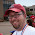## Friday, January 14, 2011

### Interfacing an Arduino with a Rate Gyro

After breadboarding an Arduino for the Sparkfun AVC RC truck robot (somebody help me name this thing) the time was ripe to tackle the LISY300AL rate gyro breakout board I bought from Sparkfun.

My hope is that this device or something like it can be used to help Pokey negotiate 90 degree turns in the firefighting maze and to help the AVC RC Truck bot execute turns and hold a heading.

The breakout board is simple.  There's 3.3V power, GND, analog voltage out, then two logic pins, one for powerdown (PD) and one for self test (ST).  Tie PD and ST to GND, and feed the thing power, then read the output.

I'd previously made a DIY, surface mount (SMT/SMD) 3.3V regulator expressly to power a rate gyro. That gyro is dead now because I accidentally fed it 9V. Oops. I was more careful this time with the new gyro.

I hooked it up to the scope and after realizing my ground line was actually hooked to +5V, I got a proper ~1.65V reading on the scope and on my DMM.

It was time to get an Arduino to read values and spit them out over serial. My breadboard Arduino came in handy, although I wished I'd used a longer breadboard as it's pretty cramped.  I wrote up some simple code to convert analog data and spit it back out over serial.


int gyroPin = 3;
float val;
float sum;

void setup() {
Serial.begin(38400);
delay(1000); // allow setup time for gyro
// try to calibrate to an average
for (i=0; i < 2048; i++) {
delay(5);
sum += (float) analogRead(gyroPin);
}
val = sum / 2048;
sum = 0;
}

void loop() {
sum += val - (float) analogRead(gyroPin);
Serial.println(sum, DEC);
delay(5);
}


The result was ... a mess.  A sine wave output with occasional, negative spikes.  How the heck was I supposed to use this data to steer a car straight?  I played around with capacitors, software averaging, all kinds of stuff, and none of it was a match for this gruesome analog signal.

Then I realized I had left PD and ST floating. I tried grounding them and...

Oh. So that was the problem. *sigh*

Now the graph holds pretty tightly to an average value with little variance.  That's some usable data. The other problem is that Vref for the ADC is 5V and the gyro outputs a max of 3.3V, so I'm losing some resolution.

Calibrating the sensor and dealing with drift are the next problems. I need to find the steady state middle point voltage put out by the sensor.  Then I can read voltage values at a fixed period, convert the voltage value to degrees/sec and integrate (add them up) to find out the current heading. Sounds easy on paper.

After some playing around to get familiar with the device and its output over time, my first experiment with the rate gyro was an attempt to study the noise level from the device while it is sitting still.

I set up simple code to track the change in output between each set of 64 samples (with 2ms delay between each).

I wrote a small perl program to generate a histogram of values and calculate the mean, variance, and standard deviation.


#!/usr/bin/perl

$i = 0; while (<>) { s/[\r\n\s]//g;$HIST{$_}++;$sum += $_;$i++;
}
$count =$i;

$avg =$sum / $i;$var = 0;
for ($i=-40;$i <= 40; $i++) { print$i, ",", $HIST{$i}, "\n";
$var +=$HIST{$i}/$count * ($i -$avg)^2;
}

$stddev = sqrt($var);

print "Average, $avg\nVariance,$var\nStdDev, \$stddev\n";


Then I graphed the histogram in the OpenOffice Calc (spreadsheet) and came up with this fairly Gaussian-looking distribution after almost 22,000 samples.

I got a mean of 411.4e-6 (which seems to be drifting a tiny bit, by the way), Var = 162 and StdDev = 12.7280. Aside from being sort of interesting and instructive for simple algorithms, I am hoping that this modeling of the sensor's uncertainty will be useful in developing a Kalman Filter when it comes time to fuse multiple sensors to get more accurate heading estimates.

I'm just starting to dig into the Kalman Filter topic but it seems that some knowledge of the noise statistics of the sensor might be useful, although manufacturers put these in their datasheets. Essentially the Kalman filter is intended to filter out measurement noise to estimate some 'true' value, like heading.

It's a bit involved and I don't pretend to understand it yet, but I gather it's sort of an iterative implementation of least squares curve fitting and is related to Bayesian statistics wherein a new but statistically noisy input is used to refine a prior estimate.

As far as I can tell you take a measurement at each fixed time step, predict where the system will be at the next time step, and use the next measurement to refine the estimate and to make a better prediction for next time step.

All the cool roboticists are doing it.

So, I'm in the middle of reading a number of papers and a book, Fundamentals of Kalman Filtering: A Practical Approach (Zarchan). I hope to know more soon.

Rest assured if I manage to learn anything, I'll share it with you.

1.I may be wrong but the code you provided seems to contain an error in Loop();

I think:
sum += val - (float)analogRead(gyroPin);

Should be:
sum = val - (float)analogRead(gyroPin);

Otherwise the output value will be an accumulation of the drift rather than a discreet output of it.

2.@Mykel -- Thanks for the note. The code is supposed to output heading versus time; it integrates the gyro value (heading rate change) over time. The val variable is intended to (crudely) calibrate for gyro offset.

Note: Only a member of this blog may post a comment.